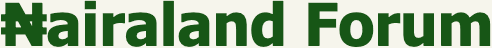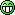#Welcome, Guest: Register On Nairaland / LOGIN! / Trending / Recent / New
Stats: 3,108,986 members, 7,672,038 topics. Date: Sunday, 10 December 2023 at 07:42 AM

## Nairaland Mathematics Clinic - Education (3) - Nairaland

 Re: Nairaland Mathematics Clinic by godstino(m): 10:18am On Jan 03, 2013 WebsiteDesigner: @Richiez, pls can u solve a Von Smoluchowski equation. Let me know if u can. Re: Nairaland Mathematics Clinic by agog: 10:19am On Jan 03, 2013 Richiez: Mathematical induction is a method of mathematically proving that a statement is true for all natural numbers i.e positive integersit usually involves 2 basic steps;1. showing that the statement is true when the lowest natural number is used e.g n=0 or n=1 (preferably)2. showing that if the statement is true for any natural number n=k, then it is true for the next natural number n=k+1let us do some examples;1. prove by mathematical induction 1+2+3+4+.......+n+(n+1) = n(n+1)/2SOLUTION note that n represents the number of elements in the seriesstep 1---we have to show that the statement is true for n=1 1= 1(1+1)/2 1= 2/2 1=1 therefore since L.H.S = R.H.S the statement is true for n=1we can even go ahead to show that the statement is true for any other small value of n, say n= 4 1+2+3+4 = 4(4+1)/2 10= 4(5)/2 10= 20/2 10= 10 therefore it is also true for n=4next is step 2here we shall consider an arbitrary value of n, say n=k and assume it is truei.e 1+2+3+4+....+k = k(k+1)/2 ................(1)if the above statement is true, then the final step is to show that it is also true for n= k+1 1+2+3+4+....+k+(k+1) = (k+1)(k+1+1)/2 1+2+3+4+....+k+(k+1) = (k+1)(k+2)/2 ...............(2)to show that eqn 2 is true, let us add (k+1) to both sides of eqn 1 1+2+3+4+....+k+(k+1) = k(k+1)/2 + (k+1).............(3)the job here is to make the R.H.S of eqn 3 look like that of eqn 2taking l.c.m as 2 1+2+3+4+....+k+(k+1) = [k(k+1)+2(k+1)]/2since (k+1) is a common factor in the R.H.S we factorize 1+2+3+4+....+k+(k+1) = (k+1)(k+2)/2This is same as eqn 2 above, hence the statement 1+2+3+4+....n+(n+1) = n(n+1)/2 is true for all natural numbers.feel free to post more exercises. I give it to you fair and square. You inspire me! 1 Like Re: Nairaland Mathematics Clinic by Ehinafe: 10:21am On Jan 03, 2013 Richiez:nice try bro, although you made some some mistakes especially at the point where you took log of both sides. e.g it ought to be log(x+y)= log5 and not logx + logy = log5. but i must admit, you did a very good job.here's another approach;x + y = 5.......(1)x^x + y^y =31.......(2)from eqn(1), x=5-y, hence we substitute this value for x in eqn(2).(5-y)^(5-y) + y^y = 31.......(3)now, we have reduced the problem to what value of y that must be inserted in eqn (3) to obtain 31.a thorough look at eqns (1) & (2) shows that the values of x & y must be positive integers within the range 0

(Go Up)

 Sections: politics (1) business autos (1) jobs (1) career education (1) romance computers phones travel sports fashion health religion celebs tv-movies music-radio literature webmasters programming techmarket Nairaland - Copyright © 2005 - 2023 Oluwaseun Osewa. All rights reserved. See How To Advertise. 138Disclaimer: Every Nairaland member is solely responsible for anything that he/she posts or uploads on Nairaland.